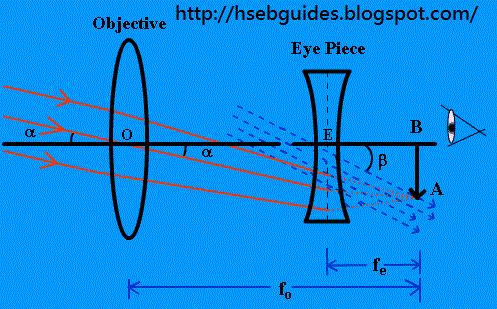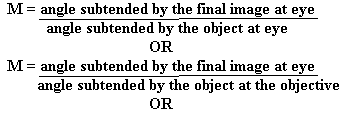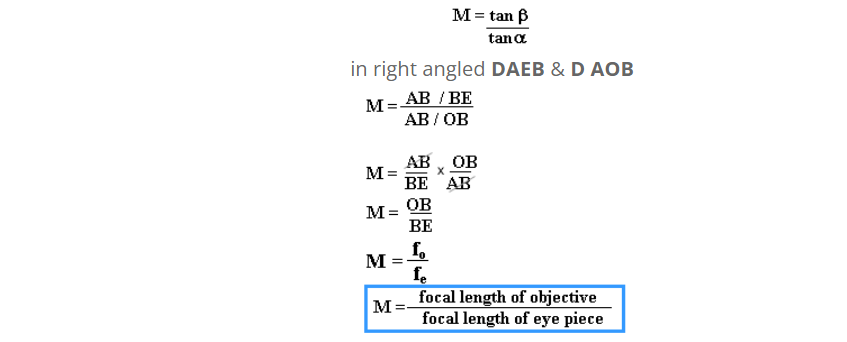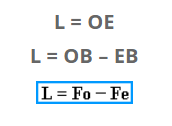# Gallilean’s Telescope

Galilieo in 1906 constructed a telescope which provides an erect image of an object only with the help of two lenses. Galilean’s telescope is used to see the object on earth.

Construction of telescope
The Galilean’s telescope consists of two lenses.

• Objective
• Eye piece

- Objective
Objective is a convex lens of large focal length “fo”

- Eye piece
Eye piece is a divergent (concave) lens of short focal length “fe“. Eye piece forms an erect and virtual image at the focus of objective on when adjustment is correct.

Working of telescope
The incident rays from a distinct object falls on the objective as a beam of parallel rays at an angle “ a ” and after refraction from objective lens these rays form an inverted image AB at its focus.
The eye piece is so adjusted that the image formed by the objective lies at a distance “fe“. The rays falling on the eye piece are refracted and emerge as parallel beam. Thus, the final image is formed at infinity.

Magnifying power of telescope
Magnifying power is the ratio of visual angle subtended by the image to the visual angle subtended by the object.
i.eIt is because the object is at infinite distance and hence the angle subtended by the object at eye may be taken as the angle subtended by the object at objective.
M = b / a

Since a and b are small angle, therefore we can assume a= tana & b = tanbThis expression shows that for large magnification focal length of objective must be larger than the focal length of eye piece.

Length of telescope
Distance b/w objective lens and eye piece is called length of telescope.
From figure:
Length of telescope is: